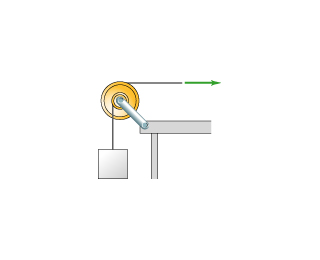# Problem: The pulley in the figure(Figure 1) represents different pulleys that are attached with outer radius and inner radius indicated in the table. The horizontal rope is pulled to the right at a constant linear speed that is the same in each case, and none of the two separate ropes slips in its contact with the pulley.A). R_outer = 0.8m R_inner = 0.4mB). R_outer = 0.2m R_inner = 0.1mC). R_outer = 0.6m R_inner = 0.5mD). R_outer = 0.4m R_inner = 0.2mE). R_outer = 0.4m R_inner = 0.3mF). R_outer = 0.6m R_inner = 0.2mRank these scenarios on the basis of the linear speed of the block.

###### FREE Expert Solution

Let the linear velocity be v.

The velocity of the block, v = (Rinner/Router)v

A) v = (0.4/0.8)v = 0.5v m/s

B) v = (0.1/0.2)v = 0.5v m/s

89% (263 ratings)###### Problem Details

The pulley in the figure(Figure 1) represents different pulleys that are attached with outer radius and inner radius indicated in the table. The horizontal rope is pulled to the right at a constant linear speed that is the same in each case, and none of the two separate ropes slips in its contact with the pulley.A). R_outer = 0.8m R_inner = 0.4m
B). R_outer = 0.2m R_inner = 0.1m
C). R_outer = 0.6m R_inner = 0.5m
D). R_outer = 0.4m R_inner = 0.2m
E). R_outer = 0.4m R_inner = 0.3m
F). R_outer = 0.6m R_inner = 0.2m

Rank these scenarios on the basis of the linear speed of the block.